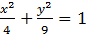### Sample Problem

Given that, find the vertices, co – vertices, and foci of the ellipse. If an answer is not a whole number, leave it as a decimal rounded to the nearest tenth.

Vertices: ( 0 , ± )

Co - vertices: ( ± , 0 )

Foci: ( 0, ± )

#### Solution

Vertices: (0, ± a ) = (0, ± 3)

Co – vertices: ( ± b, 0) = (± 2, 0)

Foci: c2=9-4=5

c = √5

≈2.2